Next: About this document ... Up: lab_template Previous: lab_template

Subsections

# Derivatives

## Purpose

The purpose of this lab is to teach you how to use Maple commands for computing derivatives.

## Background

You can compute derivatives in Maple using the limit definition of the derivative and Maple's limit command. The more common methods of computing derivative in Maple are the diff command for differentiating expressions and the D operator for differentiating functions. We will compute derivatives using all three methods.

## The limit definition of the derivative

Using the definition of derivative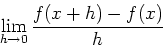, you can do this in Maple by first defining the difference quotient and the computing the limit. The following example shows how to compute the derivative ofand the evaluate the derivative at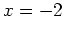.
> f:=x-> x^3;
> quot := (f(x+h)-f(x))/h;
> der:=limit(quot,h=0);
> subs(x=-2,der);

or if you don't need to see that the derivative ofisand you just want to evaluate the derivative at, then this could be done all in one Maple command as in the following example:
> f:=x->x^3;
> limit((f(-2+h)-f(-2))/h,h=0));


### The Maple D and diff commands

These commands can be summarized as follows.
• The D operator acts on a function to produce the derivative of that function.
• The diff command acts on an expression and differentiates that expression with respect to a variable specified by the user.

When you use the D operator to compute the derivative of a function, the result is also a function, as shown below.

> D(f);

If you provide a label, then you get a function you can use later in the session,
> df := D(f);

However, this is usually not necessary. See the examples below.

If you want to evaluate the derivative at a specific value of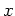or just get the expression for the derivative, you can use the following forms of the D operator.

> D(f)(2);
> D(f)(x);

This last form is the one to use for plotting, as shown below.
> plot(D(f)(x),x=-2..2);


Suppose you want to find the equaton of the line tangent to the graph of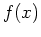at the point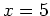. This can be done in Maple using the point slope form of a line as shown below.

> tanline := D(f)(5)*(x-5)+f(5);


The D operator cannot be used on expressions, for example trying to use it to differentiate the expresssion we defined above results in an error.

> D(p);

If you recall that Maple uses f(x) to refer to the expresssion that is used to define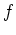, then the following error shouldn't surprise you.
> D(f(x));


To differentiate expressions, you need to use the diff command. Here is an example.

> diff(p,x);

The diff command can also be applied to functions as shown below.
> diff(f(x),x);

Note, however, that the result of the diff command is an expression, not a function. This means that computing the value of the derivative at a specific value ofrequires you to use the subs command as follows:
> der := diff(p,x);
> subs(x=Pi/2,der);


## Exercises

1. Show that the derivative of the functionisby using the limit definition of derivative.

2. Use a variable name and expression notation to define,
1. Compute the derivative and label the derivative using a variable name.
2. Evaluate the derivative at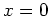,, and.
3. Plot the expression over the interval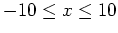. Using this plot, can you explain why the expression was not differentiable at one of thevalues given above?

3. Find the equation of the line tangent to the graph of the function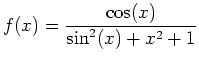at. When calculating the derivative at a point, use the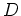command. Include a plot of the function and the tangent line on the same graph over the interval.

4. For the same function,,
1. Plotover the interval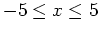and state how many horizontal tangent lines to the graph there are ONLY in this interval.
2. Plot the derivative ofover the same interval. Explain how this supports your answer above.
3. Using the fsolve command along with labels, find eachvalue where a horizontal tangent line is located. Find the corresponding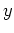values by plugging eachvalue back into the function. State in text all points on the graph ofwhere the tangent line is horizontal.Next: About this document ... Up: lab_template Previous: lab_template
Dina J. Solitro-Rassias
2008-09-17